## Year 5 maths worksheet: square numbers 1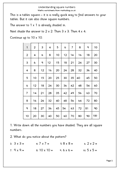This worksheet has a 10×10 multiplication square which is a brilliant aid to helping with tables, but it is also excellent for showing the pattern of square numbers from 1 to 10.

A square number is made by multiplying a whole number by itself. eg 4 x 4 = 16.

16 is a square number.

It is quite useful to learn the first 10 square numbers off by heart:

1, 4, 9, 16, 25, 36, 49, 64, 81, 100.

We will look more closely at square numbers later.

Square numbers (pg 1)

## News: More technology in schools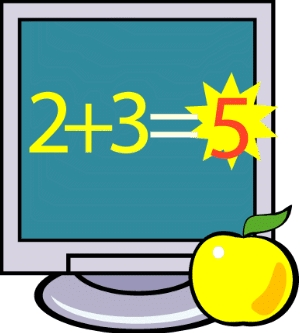Teachers are going to be given extra training so that they can keep up with their computer expert pupils. Under the latest proposals the primary timetable should be more flexible and the internet should be embedded within it. Pupils will be encouraged to use websites such as Google Earth, Wiki and Mathsblog (well why not?) to study the new Areas of Learning.

These Areas of learning are:

Understanding English, communication and languages
Mathematical understanding
Scientific and technological understanding
Human, social and environmental understanding
Understanding physical health and well-being
Understanding the arts and design.

Teachers will need to have training on the latest developments on the web and become more aware of the fantastic possibilities which are now available. Good.

## Mental arithmetic: subtracting from 100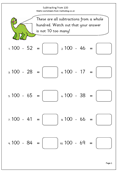Here is a maths worksheet on using mental arithmetic to subtract from 100. This is probably the type of calculation we do more than any other, especially when working out change from £1.00. There are several processes or ways to do these.

Let’s look at an example: 100 – 47.

One way is to add on 3 to make 50 then 50 to make 100: add the 50 and the 3 to make 53.

Another way is to add 50 to make 97 then count on 3 to make 100.

Watch out for the very common error that children make which is adding 3 to the 7 and adding 60 to the 40 and coming up with the answer 64!

Subtract from 100 (pg 1)

## Year 3 maths worksheet: counting in tens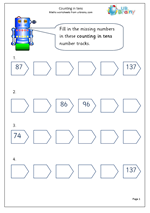This free maths worksheet is a development  in counting on in tens as it involves crossing the hundreds boundary. Most children find it easy to count on in tens until the number  becomes more than 100, then a certain amount of confusion can occur. It is a good idea to have a number line which goes across the hundred – of course most of us have a ready made one in the form of a tape measure. (As long as you use the centimetres!)

Counting in tens (pg 1)

## Year 2 maths worksheet: fractions, halves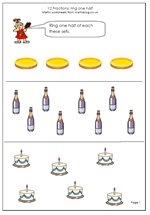This is the second in our mini-series of fractions worksheets suitable for year 2 children, aged 5/6. The first looked at taking half of an object, in other words dividing one thing into two equal parts. This worksheet looks at a different idea of a half, that is, taking half of a number of objects. Children of this age should be beginning to know, off by heart, their doubles and halves of small numbers, and this type of work will help them.
One way to do this is to pair off the objects, one at a time. Maybe make a small mark on them, although practical work is still the best way to do this. Another way is to count all the objects and then work out what half would be. With a small number of objects it is much easier to see instantly what half is. Again, it is important to show that there is more than one to show the answer. A good mini investigation would be to show the same 6 buttons several times and ask for different ways to ring half of them.

Fractions: finding one half

## News: fractions, square numbers and much moreLook out for some great free maths worksheets next week. We have a wide selection of pages coming, ranging from some early years fractions to square numbers and multiplying decimals. If that’s not enough why not try some mental arithmetic in the form of counting in tens or subtracting from 100? We have well over 500 free maths worksheets now, as well as some great maths games for the early years. make sure that you have us on your bookmarks and don’t forget to tell your friends!

## Year 5 Maths worksheet: Place value with decimals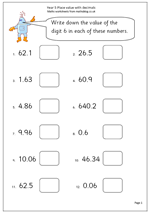By Year 5 children should have a good understanding of place value and know that the value of a digit is dependent on its place in the number. eg the 6 in 3.6 is six tenths, whilst the 6 in 16.4 is 6 units. This worksheet should demonstrate whether this concept has been understood. It asks that the value of the digit 6 is written down for each number shown, working with numbers up to to decimal places. This could be done as numbers or in words. Talk about the decimal fraction 0.6, that it can be read as six tenths or 6/10 and that 0.06 is six hundredths or 6/100.

Place value with decimals (pg 1)

## Y2 Maths worksheet: Fractions, recognising halves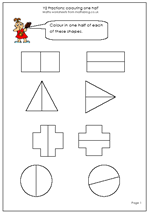An important part of early fractions work is to recognise that usually there is more than one way to colour/shade/take one half of a shape. This maths worksheet, written for year 2 children, helps with recognising halves and also demonstrates with each of the four shapes that there is more than one way to divide them into two equal parts. A similar exercise can be carried out when asking children to take half of a number of objects (buttons etc). This will follow late on another worksheet.

Fractions: recognising halves

## Year 6 Booster worksheet for SATs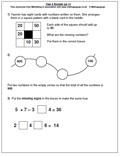Well, we are creeping towards those SAT tests so here is a contribution from MathSphere, the undoubted experts in Primary Maths.

It shows a typical looking page from the test paper and is excellent revision to ensure that children are familiar with the types of question and how to go about them.

Probably the best revision guide of all is the MathSphere Booster CD with hundreds and hundreds of pages of examples aimed at boosting children’s levels from 3 to 4 or from 4 to 5.

Booster page 12

## Multiplying by whole tens pg 1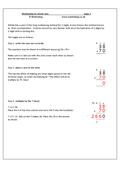This method for multiplying by whole tens follows the ‘short multiplication’ method for multiplying 2-digits by 1-digit, but is also the first step in ‘long multiplication’. Children should be very familiar with short multiplication of 2-digits by 1-digit before moving on to this work.

It also assumes a good knowledge of place value and that, by moving a digit one place to the left the number will be multiplied by 10. Therefore by multiplying by 7 and placing each digit of the answer one place to the left, the result is to multiply by 70 (Don’t forget the zero in the units).

This maths worksheet shows the method and sets out 12 questions.

Multiply by whole tens (pg 1)